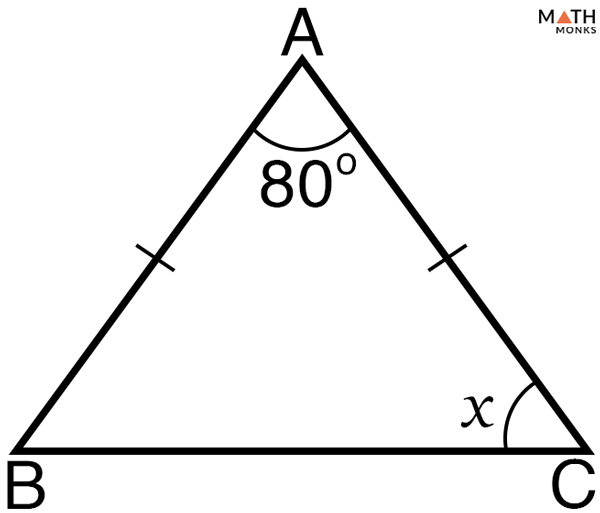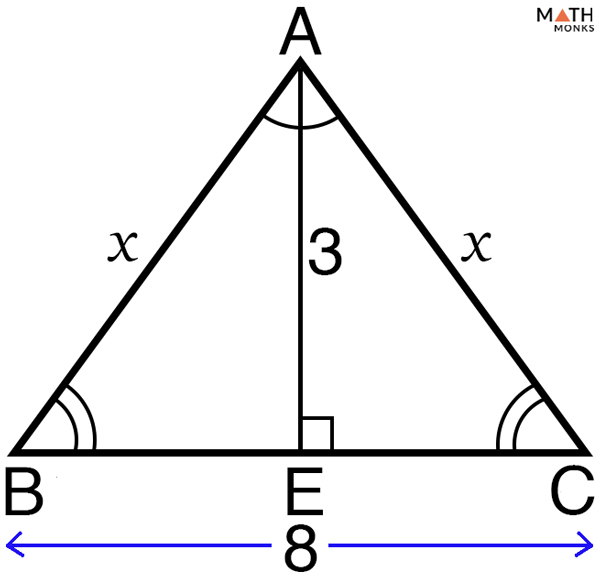# Isosceles Triangle Theorem

An isosceles triangle is a triangle having two equal sides, no matter in what direction the apex or peak of the triangle points. The key properties of isosceles triangle are:

• Contains two equal sides with the base being the unequal, third side
• The angles opposite the two equal sides are equal
• When the third angle is 90°, it is called a right isosceles triangle

Using the properties of isosceles triangle, the two theorems along with their proofs are given below.

## What is the Isosceles Triangle Theorem

Also known as the base angle theorem.

## Isosceles Triangle Theorem Proof

To prove: ∠ABC = ∠ACB

Proof:

A bisector of ∠BAC, AE is drawn

Given: AB = AC

Hence proved that, the angles opposite to the equal sides of an isosceles triangle are also equal.

## What is the Converse of the Isosceles Triangle Theorem

### Converse of the Isosceles Triangle Theorem Proof

To prove: AB = AC

Proof:

A bisector of ∠BAC, AE is drawn that meets BC at right angles

Given: ∠ABC = ∠ACB

Hence proved that, the sides opposite to the two equal angles of a triangle are equal.

## Isosceles Triangle Theorem Examples

Let us solve some problems to understand the theorems better.Find the value of x in the given isosceles triangle.

Solution:

As we know, according to the isosceles triangle theorem,
∠ACB = ∠ABC = x
Now, by the triangle sum theorem,
∠ABC + ∠ACB + ∠BAC = 180°
x + x + 80° = 180°
2x = 180° – 80° = 100°
x = 50°

In ∆XYZ, XY = XZ, and ∠XYZ = 55°. Find ∠YXZ.

Solution:

As we know, according to the isosceles triangle theorem,
∠XYZ = ∠XZY = 55°
Now, by the triangle sum theorem,
∠XYZ + ∠XZY + ∠YXZ = 180°
55° + 55° + ∠YXZ = 180°
∠YXZ = 180° – (55° + 55°)
∠YXZ = 180° – 110°
∠YXZ = 70°Solve the value of x in the given isosceles triangle.

Solution:

Here we will use the Pythagorean Theorem to find the side lengths of the given isosceles triangle.
Since AE divides BC into BE and EC
Thus,
BE = EC = 4cm
Applying Pythagorean Theorem in ∆ABE,
AB2 = AE2 + BE2, here AE = 3, BE = 4
x2 = (3)2 + (4)2
x2 = 9 + 16 = √25
x = 5 units
Again, since ∆ABC is an isosceles triangle, with sides AB = AC
Hence, AC = x = 5 units